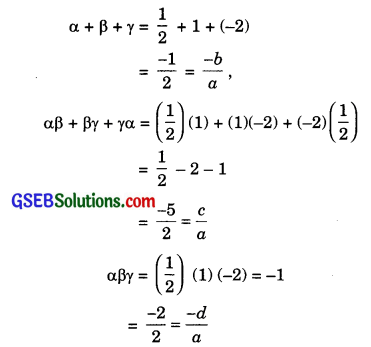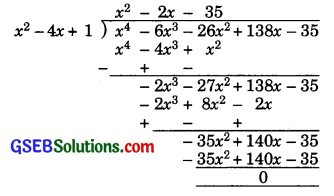# GSEB Solutions Class 10 Maths Chapter 2 Polynomials Ex 2.4

Gujarat Board GSEB Solutions Class 10 Maths Chapter 2 Polynomials Ex 2.4 Textbook Questions and Answers.

## Gujarat Board Textbook Solutions Class 10 Maths Chapter 2 Polynomials Ex 2.4

Question 1.
Verify that the numbers given alongside of the cubic polynomials below are their zeroes. Also verify the relationship between the zeroes and the coefficients in each case:

1. 2x3 + x2 – 5x + 2; $$\frac{1}{2}$$, 1, -2
2. x3 – 4x2 + 5x – 2; 2, 1, 1

Solution:
1. Let p(x) = 2x3 + x2 – 5x + 2
Then, we have
$$p\left(\frac{1}{2}\right)=2\left(\frac{1}{2}\right)^{3}+\left(\frac{1}{2}\right)^{2}-5\left(\frac{1}{2}\right)+2$$
= $$\frac{1}{4}+\frac{1}{4}-\frac{5}{2}+2=0$$
p(1) = 2(1)3 + (1)2 – 5(1) + 2
= 2 + 1 – 5 + 2 = 0
and, p(-2) = 2(-2)3 + (-2)2 – 5(-2) + 2
= -16 + 4 + 10 + 2 = 0
Therefore, $$\frac{1}{2}$$, 1 and -2 are the zeroes of 2x3 + x2 – 5x + 2.
Comparing the given polynomial with ax3 + bx2 + cx + d, we get
a = 2
b = 1
c = -5
d = 2
Let α = $$\frac{1}{2}$$
β = 1
and γ = -2
Then, we have2. Let p(x) = x3 – 4x2 + 5x – 2
Then, we have
p(2) = (2)3 – 4(2)2 + 5(2) – 2
= 8 – 16 + 10 – 2 = 0
p(1) = (1)3 – 4(1)2 + 5(1) – 2
= 1 – 4 + 5 – 2 = 0
Therefore, 2 and 1 are the two zeroes of x3 – 4x2 + 5x – 2. Hence (x – 2) (x – 1), i.e., x2 – 3x + 2 is a factors of the given polynomial. Now, we apply the division algorithm to the given polynomial and x2 – 3x + 2.So, x3 – 4x2 + 5x – 2
= (x2 – 3x + 2) (x – 1)
⇒ x3 – 4x2 + 5x – 2 = (x – 2) (x – 1) (x – 1)
Hence, 2, 1 and 1 are the zeros of x3 – 4x2 + 5x – 2.
Comparing the given polynomial with ax3 + bx2 + cx + d, we get
a = 1
b = -4
c = 5
d = – 2
Let α = 2
β = 1
and γ = 1
Then, we have
α + β + γ = 2 + 1 + 1 = 4
= –$$\frac{-4}{1}$$ = –$$\frac{b}{a}$$
αβ + βγ + αγ = (2)(1) + (1)(1) + (1)(2)
= 5 = $$\frac{5}{1}$$ = $$\frac{c}{a}$$
αβγ = (2) (1) (1) = 2
= –$$\frac{-2}{1}$$ = $$\frac{-d}{a}$$Question 2.
Find a cubic polynomial with the sum, sum of the product of its zeros taken two at a time, and the product of its zeroes as 2, -7, -14 respectively.
Solution:
Let the cubic polynomial be ax3 + bx2 + cx + d and its zeroes be α, β and γ.
Then, α + β + γ = 2= $$\frac{-b}{a}$$
αβ + βγ + γα = -7 = $$\frac{c}{a}$$
αβγ = -14 = $$\frac{-d}{a}$$
If a = 1, then b = -2, c = -7 and d = 14.
Hence, one cubic polynomial which fits the given conditions is
x3 – 2x2 – 7x + 14.

Question 3.
If the zeroes of the polynomial
x3 – 3x2 + x + 1 are a – b, a, a + b, find a and b.
Solution:
Then given polynomial is x3 – 3x2 + x + 1
Comparing with Ax3 + Bx2 + Cx + D, we get
A = 1
B = -3
C = 1
D = 1
Let α = a – b
β = a
γ = a + b
Then, we have
α + β + γ = $$\frac{-B}{A}=-\frac{(-3)}{1}=3$$
⇒ a – b + a + a + b = 3
⇒ 3a = 3
⇒ a = 1
αβγ = $$\frac{-D}{A}$$ = -1
⇒ (a – b) a (a + b) = -1
⇒ (1 – b) 1(1 + b) = -1
⇒ 1 – b2 = -1
⇒ b2 = 2
⇒ b = ± $$\sqrt { 2 }$$Question 4.
If two zeroes of the polynomial x4 – 6x3 – 26x2 + 138x – 35 are 2 ± $$\sqrt { 3 }$$, find the other zeroes.
Solution:
Since two zeroes of the given polynomial x4 – 6x3 – 26x2 + 138x – 35 are 2 ± $$\sqrt { 3 }$$, therefore,
(x – (2 + $$\sqrt { 3 }$$)) (x – (2 – $$\sqrt { 3 }$$)),
i.e., ((x – 2) – $$\sqrt { 3 }$$) (x – 2) + $$\sqrt { 3 }$$)
i.e., (x – 2)2 – ($$\sqrt { 3 }$$)2, i.e., x2 – 4x + 1
is a factor of the given polynomial. Now, we apply the division algorithm to the given polynomial and x2 – 4x + 1.So, x4 – 6x3 – 26x2 + 138x – 35
= (x2 – 4x + 1) (x2 – 2x – 35)
= (x2 – 4x + 1) (x – 7) (x + 5)
Hence, the other two zeroes are 7 and -5.Question 5.
If the polynomial x4 – 6x3 + 16x2 – 25x + 10 is divided by another polynomial x2 – 2x + k, the remainder comes out to be x + a, find k and a.
Solution:
Let us apply the division algorithm to the give Polynomial x4 – 6x3 + 16x2 – 25x + 10 and another Polynomial x2 – 2x + k.Remainder
= (2k – 9)x – k (8 – k) + 10
But the remainder is given to be x + a.
Therefore, 2k – 9 = 1
⇒ 2k = 10 ⇒ k = 5
and -k(8 – k) + 10 = a
⇒ -5(8 – 5) + 10 = a
⇒ – 5 = a
⇒ a = -5